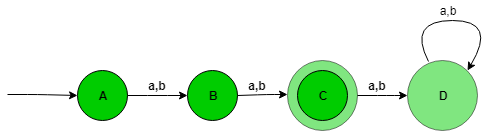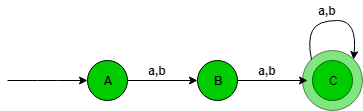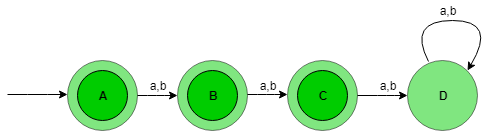GeeksforGeeks App
Open AppBrowser
Continue

# Designing Deterministic Finite Automata (Set 1)

Prerequisite – Designing finite automata In this article, we will see some designing of Deterministic Finite Automata (DFA).

Problem-1: Construction of a DFA for the set of string over {a, b} such that length of the string |w|=2 i.e, length of the string is exactly 2. Explanation – The desired language will be like:

`L = {aa, ab, ba, bb} `

The state transition diagram of the language will be like:Here, State A represent set of all string of length zero (0), state B represent set of all string of length one (1), state C represent set of all string of length two (2). State C is the final state and D is the dead state it is so because after getting any alphabet as input it will not go into final state ever.

```Number of states: n+2
Where n is |w|=n ```

The above automata will accept all the strings having the length of the string exactly 2. When the length of the string is 1, then it will go from state A to B. When the length of the string is 2, then it will go from state B to C and when the length of the string is greater than 2, then it will go from state C to D (Dead state) and after it from state D TO D itself.

## Python3

 `#check string in``#in state A``def` `checkStateA(n):``    ` `    ``#if length of``    ``#string is one``    ``#print not accepted``    ``if``(``len``(n)``=``=``1``):``        ``print``("string ``not` `accepted")``    ``else``:  ``        ``#pass string to stateB to``        ``#to check further transitions``        ``if``(n[``0``]``=``=``'a'` `or` `n[``0``]``=``=``'b'``):``            ``stateB(n[``1``:])``            ` `            ` `def` `stateB(n):``    ``#here if length``    ``#is not 1 print#string not accepted``    ``if``(``len``(n)!``=``1``):``        ``print``("string ``not` `accepted")``    ``else``:``        ``#else pass string``        ``#to state c``        ``stateC(n[``1``:])``def` `stateC(n):``    ``#here if length``    ``#becomes zero``    ``#print accepted``    ``#else not accepted``    ``if` `(``len``(n)``=``=``0``):``        ``print``("string accepted")``    ``else``:``        ``print``("string ``not` `accepted")``    ` `    ` `#take input   ``n``=``input``()``checkStateA(n)`

Problem-2: Construction of a DFA for the set of string over {a, b} such that length of the string |w|>=2 i.e, length of the string should be at least 2. Explanation – The desired language will be like:

`L = {aa, ab, ba, bb, aaa, aab, aba, abb........} `

The state transition diagram of the language will be like:Here, State A represent set of all string of length zero (0), state B represent set of all string of length one (1), and state C represent set of all string of length two (2).

```Number of states: n+1
Where n is |w|>=n ```

The above automata will accept all the strings having the length of the string at least 2. When the length of the string is 1, then it will go from state A to B. When the length of the string is 2, then it will go from state B to C and lastly when the length of the string is greater than 2, then it will go from state C to C itself.

## Python3

 `#check string in``#in state A``def` `checkStateA(n):``    ` `    ``#if length of``    ``#string is one``    ``#print not accepted``    ``if``(``len``(n)``=``=``1``):``        ``print``("string ``not` `accepted")``    ``else``:  ``        ``#pass string to stateB to``        ``#to check further transitions``        ``if``(n[``0``]``=``=``'a'` `or` `n[``0``]``=``=``'b'``):``            ``stateB(n[``1``:])``            ` `            ` `def` `stateB(n):``    ` `    ``#here if length``    ``#is less than 1``    ``#printstring not accepted``    ``if``(``len``(n)<``1``):``        ``print``("string ``not` `accepted")``    ``else``:``        ` `        ``#else pass string``        ``#to state c``        ``stateC(n[``1``:])``        ` `        ` `def` `stateC(n):``    ``#here if length of string``    ``#is greater than equal to zero``    ``#print accepted``    ``#else not accepted``    ``if` `(``len``(n)>``=``0``):``        ``print``("string accepted")``    ``else``:``        ``print``("string ``not` `accepted")``    ` `    ` `#take input   ``n``=``input``()``checkStateA(n)`

Problem-3: Construction of a DFA for the set of string over {a, b} such that length of the string |w|<=2 i.e, length of the string is atmost 2.
Explanation – The desired language will be like:

`L = {?, aa, ab, ba, bb} `

The state transition diagram of the language will be like:Here, State A represent set of all string of length zero (0), state B represent set of all string of length one (1), state C represent set of all string of length two (2), state A, B, C is the final state and D is the dead state it is so because after getting any alphabet as input it will not go into final state ever.

```Number of states: n+2
Where n is |w|<=n ```

The above automata will accept all the strings having the length of the string at most 2. When the length of the string is 1, then it will go from state A to B. When the length of the string is 2, then it will go from state B to C and lastly when the length of the string is greater than 2, then it will go from state C to D (Dead state).

## Python3

 `#check string in``#in state A``def` `checkStateA(n):``    ` `    ``#if only two transition occurs``    ``#then print string accepted``  ` `    ``if``(n[``0``]``=``=``'a'` `or` `n[``0``]``=``=``'b'``):``        ``stateB(n[``1``:])``            ` `            ` `def` `stateB(n):``    ` `    ``#if length is 0``    ``#print accepted``    ``if``(``len``(n)``=``=``0``):``        ``print``("string accepted")``    ``else``:``        ``stateC(n[``1``:])``        ` `        ` `def` `stateC(n):``    ``#if length is 0``    ``#print accepted``    ``#else not accepted``    ``if` `(``len``(n)``=``=``0``):``        ``print``("string accepted")``    ``else``:``        ``print``("string ``not` `accepted")``    ` `    ` `#take input   ``n``=``input``()``checkStateA(n)`

My Personal Notes arrow_drop_up